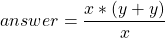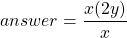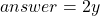# Programming operations, using maths

## Introduction

An important part of computer programming is implementing mathematic operations. Maths can be used to implement many functions in an application; be it to include functionality that is obviously maths related, or to implement functions that may not immediately appear, to the user, as a maths problem; such as calculating the window size to maintain the correct aspect ratio, or to calculate the width of a field that is to be half the width of the window.

Maths is just maths, operations in programming languages work in the same way they would on a calculator, or if the operation was written on paper.

### List of operators

Below is a list of the most used maths operators in programming languages such as PHP & Java.

Arithmetic
Subtraction
* Multiplication
/ Division
% Remainder
++ Increment
Decrement
( ) Order of operation
Relation
== Equal to
!= Not equal to
> Greater than
< Less than
>= Greater than or equal to
<= Less than or equal to
Conditions
& & And (Both conditions must be true)
|| Or (Either condition must be true)

## Examples

### Java

class MathsExample()
{

public MathsExample()
{

}

public float arithmeticExample(float firstNumber, float secondNumber)
{
answer = firstNumber * (secondNumber + secondNumber) / firstNumber;
}

public int relationExample(float firstNumber, float secondNumber)
{
if (firstNumber > secondNumber)
{
return 1;
}
else if (firstNumber == secondNumber)
{
return 0;
}
else
{
return -1;
}
}

public boolean conditionExample(float firstNumber, float secondNumber)
{
if (firstNumber > secondNumber || secondNumber > firstNumber)
{
return true;
}
else
{
return false;
}
}
}

The above MathsExample class contains three functions that perform basic maths operations and return an answer depending on the result of the operations.

The first function arithmeticExample takes two float (decimal numbers) as arguments and return s a float using the following formulae:

(1)Simplified to:

(2)Or simplified further:

(3)The second function provides an example of using relational operators. The function takes two numbers as arguments and returns ‘1’ if the firstNumber is greater than the secondNumber; ‘0’ if the firstNumber is equal to the secondNumber; or ‘-1’ otherwise (which will be if the firstNumber is less than the secondNumber.

The final function shows how conditions can be used to calculate the truth value of multiple operations in one go. If two relation operations are presented either side of ‘&&’, both operations must return true to continue; likewise if two operations are placed either side of ‘||’, either or both operations may return true to continue.

In this example the function takes two numbers as arguments and returns true if the firstNumber is greater than the secondNumber, or the secondNumber is greater than the firstNumber. You may be fooled into thinking that this operation will always return true as the firstNumber is always going to be greater than or less than the second number; in the case that both numbers are the same, this operation will of course return false. This could be simplified by using the following code instead:

if (firstNumber != secondNumber)
{
return true;
}
else
{
return false;
}

This simplified version of the code returns true if the firstNumber is not equal to the secondNumber; otherwise it returns false.

## Conclusion

Hopefully from this article you will have gained or improved on your knowledge of the following areas:

• How arithmetic is dealt with in computer programming
• How relational operations are dealt with in computer programming
• How conditional operations are dealt with in computer programming

### 5 tags for "Programming operations, using maths"

This site uses Akismet to reduce spam. Learn how your comment data is processed.